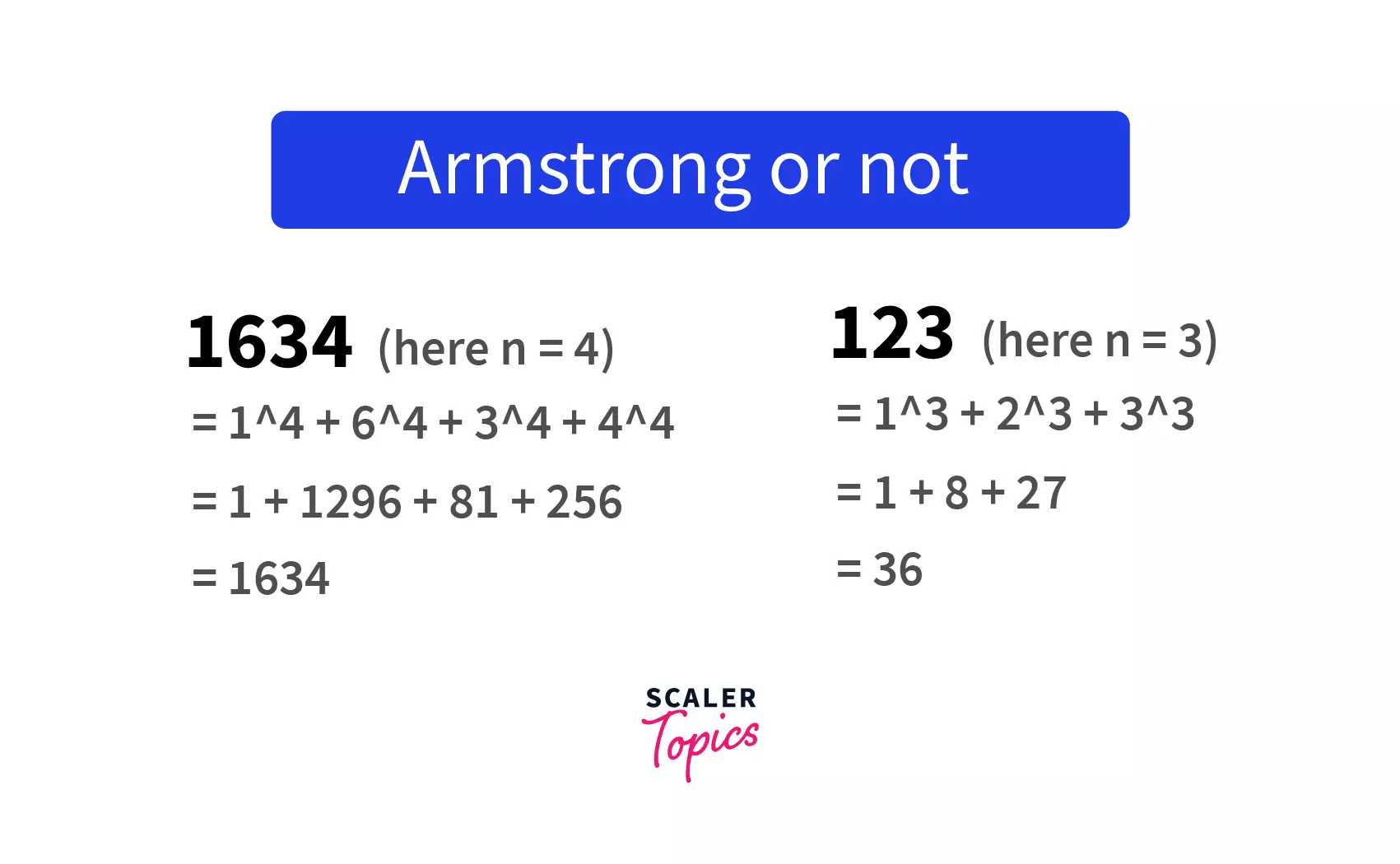# Python Program to Check Armstrong NumberChallenge Inside! : Find out where you stand! Try quiz, solve problems & win rewards!

## Learn via video courseFree Python Certification Course: Master the essentials
By Rahul JanghuFree
4.90Enrolled: 35123Free Python Certification Course: Master the essentials
Rahul JanghuFree
4.90
Enrolled: 35123

## Overview

A number of n-digits can be defined as an Armstrong number if and only if the sum of its digits powered to n is equal to the number itself. For example, 1634 is an Armstrong number as

$1634 = 1^4 +6^4 +3^4 + 4^4$

## What is Armstrong Number in Python?

The Armstrong number in Python is the number in which the sum of each digit powered to the total number of digits is the same as the given number. i.e. for a given number say $153, 1^3 + 5^3 + 3^3$ is equal to $153$.

Let's take another example; say $1634 = 1^4 +6^4 +3^4 + 4^4$ is an Armstrong number. Any number in any number system that exhibits this sum property will be called an Armstrong number.

Armstrong numbers are also called Narcissistic numbers. They don’t have real-life applications.## Algorithm to Find a 3-digit Armstrong Number in Python

To find a 3-digit Armstrong number in Python, we start by extracting each digit in the number, multiplying it thrice (to calculate its cube), and then adding all those cubes of digits.

Now, we will compare the sum with the given number. If the sum of cubes is the same as the actual number, then we call it an Armstrong number; otherwise, it is not an Armstrong number.

Step 2: Declare a variable sum and initialize it to 0

Step 2: For each digit in the number, multiply it thrice and add it to the sum.

Step 3: Check if the sum equals the initial number.

Step 4: If both are equal, then print it as an Armstrong number. Else, print it as not an Armstrong number.

Code:

Output:

## Algorithm to Find an n-digit Armstrong Number in Python

To find if an n-digit number is an Armstrong number or not, we will first calculate the number of digits that is equal to n.

Then we will extract each digit in the number and multiply it n times by itself (to calculate the nth power of it ) and add all those numbers to the sum variable.

Now, we will check if the sum is equal to the given number or not. If both are equal, then we will print it as an Armstrong number or print it as not an Armstrong number.

Step 1: Take the input

Step 2: Calculate the number of digits and store it as digits

Step 3: Declare a variable sum and initialize to 0

Step 4: For each digit in the number, multiply the same digit for digits times and then add it to the sum.

Step 5: Check whether the sum is equal to the input number.

Step 6: Print it as an Armstrong number if it is equal. Else print, not an Armstrong number.

## Python Program to Find an n-digit Armstrong Number

### Using While Loop

Code:

Output:

Explanation:

$1^4+6^4+3^4+4^4 = 1634$

As the sum of digits powered to the number of digits is equal to the number itself, the given number is an Armstrong number.

### Using Functions

Code:

Output:

Explanation:

$1^4+6^4+3^4+4^4 = 1634$

As the sum of digits powered to the number of digits is equal to the number itself, the given number is an Armstrong number.

### Using Recursion

Code:

Output:

Explanation:

$1^4+6^4+3^4+4^4 = 1634$

As the sum of digits powered to the number of digits is equal to the number itself, the given number is an Armstrong number.

## Conclusion

• We learned about what Armstrong numbers are.
• We also saw how to identify if a number is an Armstrong number in Python or not.
• We also learned various algorithms and their implementation to check whether the given number is an Armstrong number.